# Introduction to gghighlight: Highlight ggplot's Lines and Points with Predicates

## October 6, 2017 by Hiroaki Yutani

(Update: The functions introduced here is deprecated now. Please use gghighlight(), which is far nicer one)

Suppose we have a data that has too many series like this:

set.seed(2)
d <- purrr::map_dfr(
letters,
~ data.frame(idx = 1:400,
value = cumsum(runif(400, -1, 1)),
type = .,
stringsAsFactors = FALSE))


For such data, it is almost impossible to identify a series by its colour as their differences are so subtle.

library(ggplot2)

ggplot(d) +
geom_line(aes(idx, value, colour = type))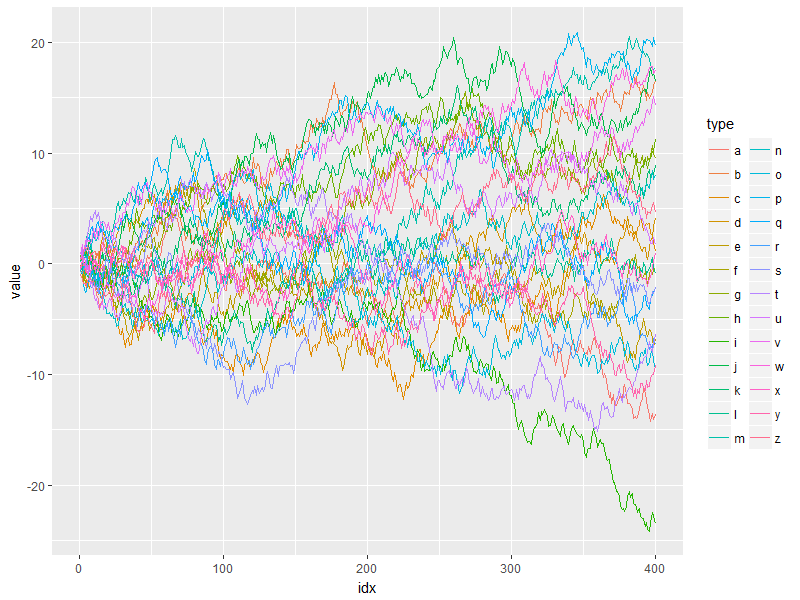## Highlight lines with ggplot2 + dplyr

So, I am motivated to filter data and map colour only on that, using dplyr:

library(dplyr, warn.conflicts = FALSE)

d_filtered <- d %>%
group_by(type) %>%
filter(max(value) > 20) %>%
ungroup()

ggplot() +
# draw the original data series with grey
geom_line(aes(idx, value, group = type), data = d, colour = alpha("grey", 0.7)) +
# colourise only the filtered data
geom_line(aes(idx, value, colour = type), data = d_filtered)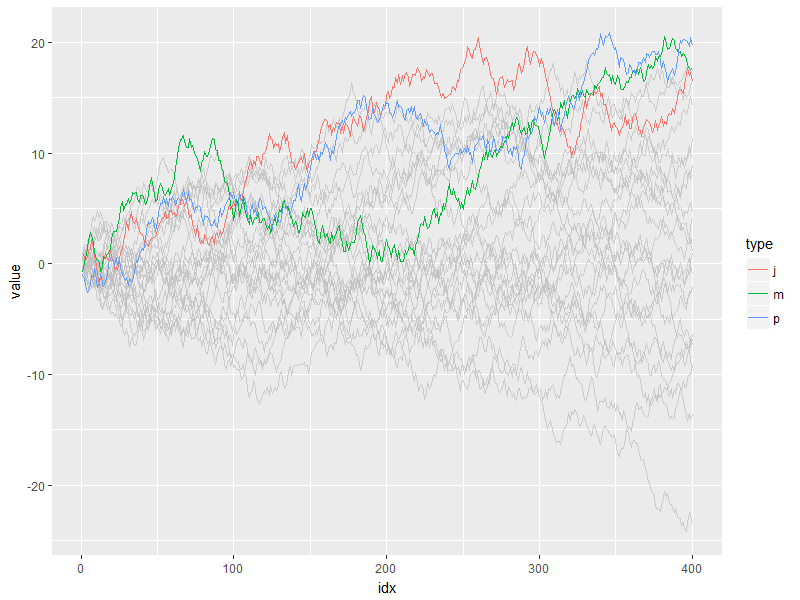But, what if I want to change the threshold in predicate (max(.data\$value) > 20) and highlight other series as well? It’s a bit tiresome to type all the code above again every time I replace 20 with some other value.

## Highlight lines with gghighlight

gghighlight package provides two functions to do this job. You can install this via CRAN (or GitHub)

install.packages("gghighlight")


gghighlight_line() is the one for lines. The code equivalent to above (and more) can be this few lines:

library(gghighlight)

gghighlight_line(d, aes(idx, value, colour = type), predicate = max(value) > 20)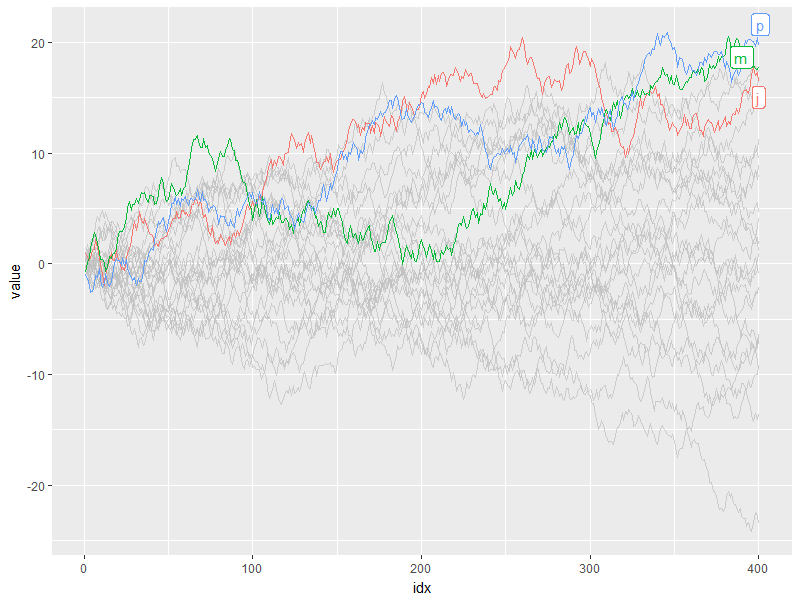As gghighlight_*() returns a ggplot object, it is fully customizable just as we usually do with ggplot2 like custom themes and facetting.

library(ggplot2)

gghighlight_line(d, aes(idx, value, colour = type), max(value) > 20) +
theme_minimal()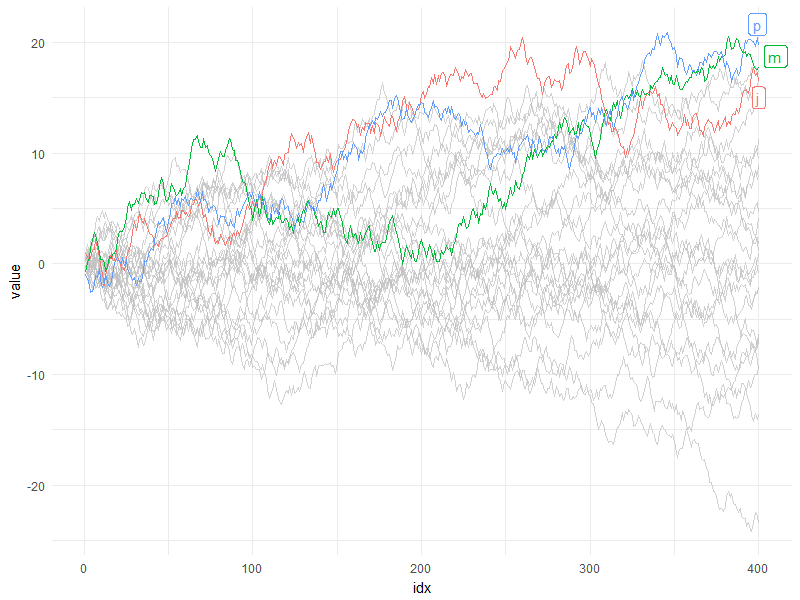gghighlight_line(d, aes(idx, value, colour = type), max(value) > 20) +
facet_wrap(~ type)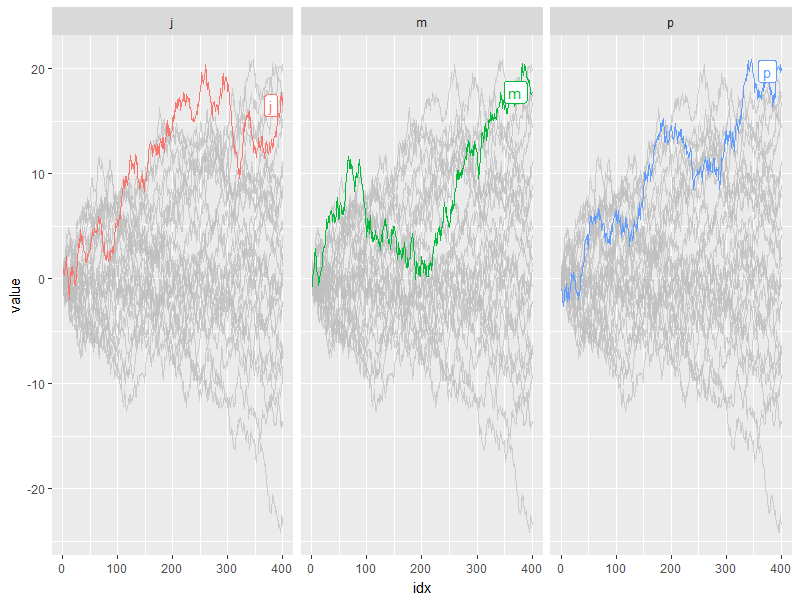By default, gghighlight_line() calculates predicate per group, more precisely, dplyr::group_by() + dplyr::summarise(). So if the predicate expression returns multiple values per group, it ends up with an error like this:

gghighlight_line(d, aes(idx, value, colour = type), value > 20)
#> Error in summarise_impl(.data, dots): Column predicate.......... must be length 1 (a summary value), not 400


## Highlight points with gghighlight

gghighlight_point() highlight points. While gghighlight_line() evaluates predicate by grouped calculation (dplyr::group_by()), by default, this function evaluates it by ungrouped calculation.

set.seed(19)
d2 <- sample_n(d, 100L)

gghighlight_point(d2, aes(idx, value), value > 10)
#> Warning in gghighlight_point(d2, aes(idx, value), value > 10): Using type
#> as label for now, but please provide the label_key explicity!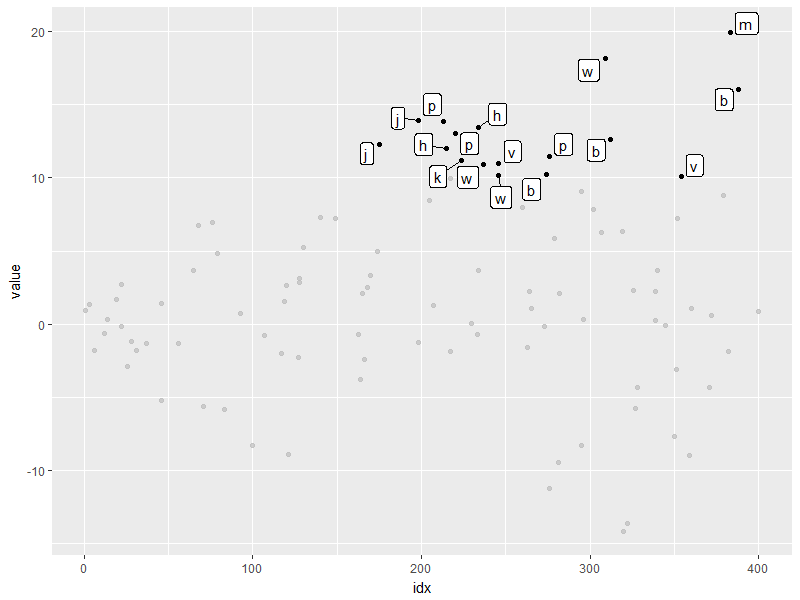As the job is done without grouping, it’s better to provide gghighlight_point() a proper key for label, though it tries to choose proper one automatically. Specifying label_key = type will stop the warning above:

gghighlight_point(d2, aes(idx, value), value > 10, label_key = type)


You can control whether to do things with grouping by use_group_by argument. If this set to TRUE, gghighlight_point() evaluate predicate by grouped calculation.

gghighlight_point(d2, aes(idx, value, colour = type), max(value) > 15, label_key = type,
use_group_by = TRUE)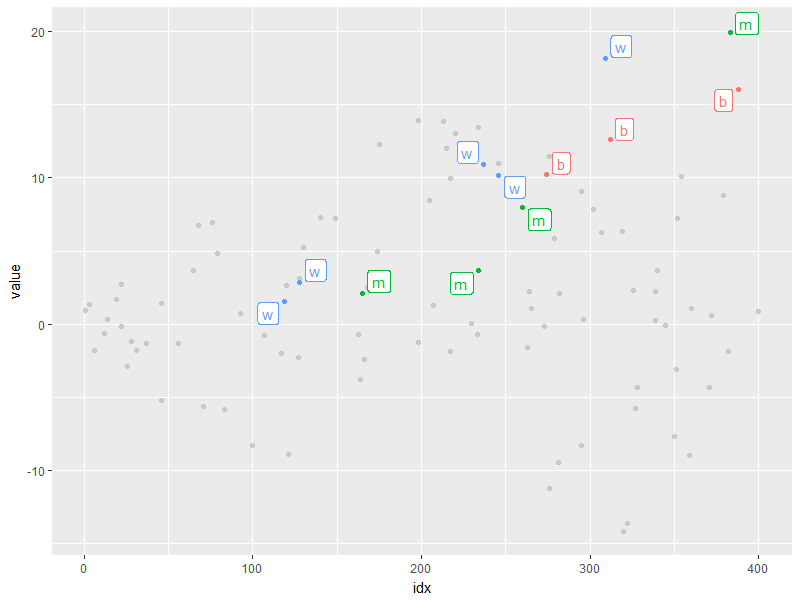## Non-logical predicate

(Does “non-logical predicate” make sense…? Due to my poor English skill, I couldn’t come up with a good term other than this. Any suggestions are wellcome.)

By the way, to construct a predicate expression like bellow, we need to determine a threshold (in this example, 20). But it is difficult to choose a nice one before we draw plots. This is a chicken or the egg situation.

max(value) > 20


So, gghiglight_*() allows predicates that will be evaluated into non-logical values. The result value will be used to sort data, and the top max_highlight data points/series will be highlighted. For example:

gghighlight_line(d, aes(idx, value, colour = type), predicate = max(value),
max_highlight = 6)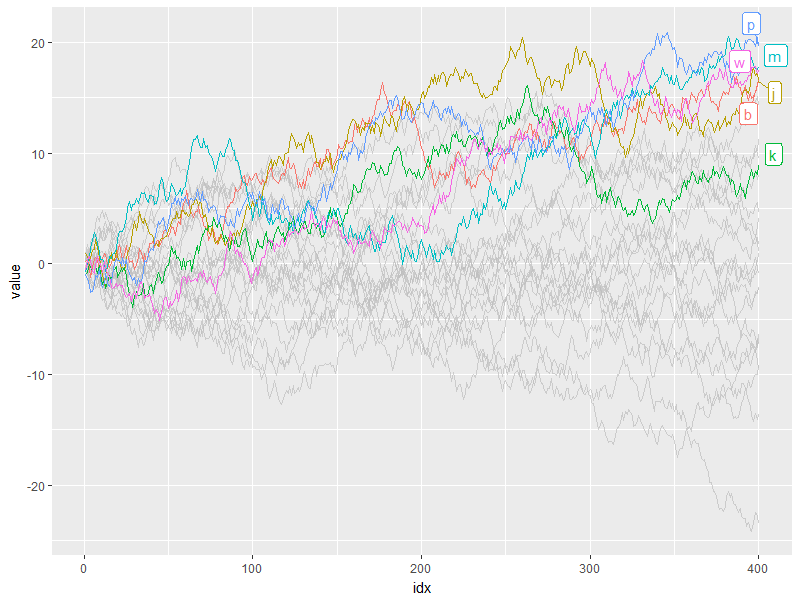## Caveats

Seems cool? gghighlight is good to explore data by changing a threshlold little by little. But, the internals are not so efficient, as it does almost the same calculation everytime you execute gghighlight_*(), which may get slower when it works with larger data. Consider doing this by using vanilla dplyr to filter data.

## Summary

gghighlight package is a tool to highlight charactaristic data series among too many ones. Please try!

Bug reports or feature requests are welcome! -> https://github.com/yutannihilation/gghighlight/issues